# Almost Periodic Function

Also found in: Wikipedia.
The following article is from The Great Soviet Encyclopedia (1979). It might be outdated or ideologically biased.

## Almost Periodic Function

a function whose value is approximately repeated when its argument is increased by properly selected constants (the almost periods). More precisely, a continuous function f(x) defined for all real values of 5 x is called almost periodic if for every > 0 there exists an l = l (∊) such that in every interval of length l on the x-axis at least one number τ = τ(∊) can be found for which the inequality ǀf (x + τ) – f(x)ǀ < ∊ is satisfied for all x. The numbers τ are called the almost periods of the function f(x). Periodic functions are special cases of almost periodic functions; simple examples of almost periodic functions that are not periodic can be obtained by adding periodic functions with incommensurable periods—for example, cos x + cos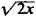.

The following are some important properties of almost periodic functions:

(1) An almost periodic function is bounded and uniformly continuous on the entire x-axis.

(2) The sum and product of a finite number of almost periodic functions is an almost periodic function.

(3) The limit of a uniformly convergent sequence of almost periodic functions is an almost periodic function.

(4) Every almost periodic function has a mean value (over the entire x-axis):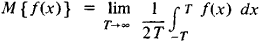(5) To every almost periodic function we can associate a Fourier series: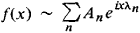where λ1, λ2, …, λn, … can be any sequence of distinct real numbers and

An = M {f (x)enx}

(6) The Parseval equality: for every almost periodic function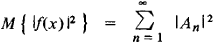(7) Uniqueness theorem: if f(x) is a continuous almost periodic function and if for all real λ

M {f (x)eiλx} = 0

then f(x) ≡ 0. In other words, a Fourier series uniquely determines an almost periodic function.

(8) Approximation theorem: for every ∊ > 0, there exists a finite trigonometric polynomial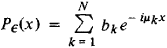(where μκ is a real number) such that the inequality ǀf(x) – P (x)ǀ < ∊ is satisfied for all values of x; conversely, every function f(x) with this property is an almost periodic function.

The first construction of almost periodic functions was given by the Danish mathematician H. Bohr in 1923. Even earlier, in 1893, the Latvian mathematician P. Bohl studied a special case of almost periodic functions—quasi-periodic functions. A new construction of the theory of almost periodic functions was provided by N. N. Bogoliubov in 1930. The theory of almost periodic functions was generalized to include discontinuous functions first by V. V. Stepanov in 1925 and subsequently by H. Weyl and A. S. Besicovitch. A generalization of a different kind was given by the Soviet mathematician B. M. Levitan in 1938.

### REFERENCES

Bohr, H. Pochti periodicheskie funktsii. Moscow-Leningrad, 1934. (Translated from German.)
Levitan, B. M. Pochti-periodicheskie funktsii. Moscow, 1953.
References in periodicals archive ?
The almost periodic function introduced seminally by Bohr in 1925 plays an important role in describing the phenomena that are similar to the periodic oscillations which can be observed frequently in many fields, such as celestial mechanics, nonlinear vibration, electromagnetic theory, plasma physics, engineering, and ecosphere.
Let us recall that any asymptotically almost periodic function is also asymptotically Stepanov p-almost periodic (1 [less than or equal to] p < [infinity]).
A function f [member of] M(R,R) is said to be a Stepanov-like asymptotical almost periodic function if it can be expressed as
A function f : T x X [right arrow] X is called an almost periodic function in t [member of] T uniformly for x [member of] X if the e-translation set of f
where [phi] = [([[phi].sub.1], [[phi].sub.2], ..., [[phi].sub.n]).sup.T] is an arbitrary almost periodic function. Based on system (37) and by using the exponential dichotomy of linear system, the following mapping was established:
Actually, the sum of the series (3.1) is an almost periodic function in the sense of Bohr, belonging to the space AP1(R, C).
First, let us introduce the notion of almost periodic function used throughout this paper.
Since [^.[mu].sub.n]([[lambda].sub.j](n)) are uniformly bounded by [MATHEMATICAL EXPRESSION NOT REPRODUCIBLE IN ASCII] the left hand side is an almost periodic function. Because [a.sub.j](n) [not equal to] 0 for all j, n, this implies [^.[[mu]].sub.nj](n)) = 0.
Then the decomposition of a weighted pseudo almost periodic function f = g + [phi] where g [member of] AP(T, X) and [phi] [member of] PA[P.sub.0](T, X, [rho]) is unique.
From the properties of almost periodic function there exists a sequence {[t.sub.n]} with [t.sub.n] [right arrow] +[infinity] as n [right arrow] +[infinity] such that
By the classical theory, F is an usual almost periodic function. Then by

Site: Follow: Share:
Open / Close